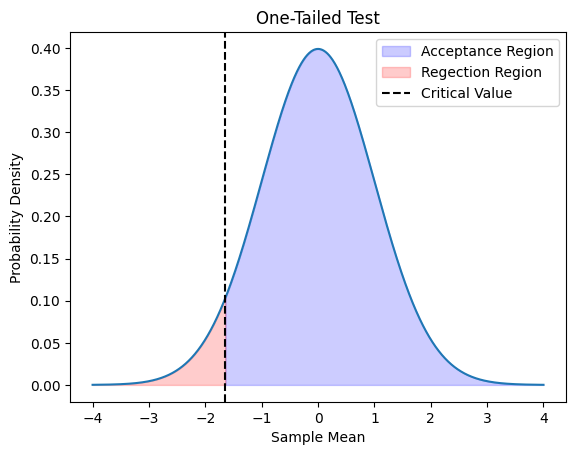Course Content

Probability Theory Mastering

##What is Statistic Hypothesis? Type 1 and Type 2 Errors

Statistical hypothesis H is an assumption about the type of distribution of the general population, which is tested on the available samples. If the distribution of the general population is known and it is necessary to check the assumption regarding the values of the distribution parameters using the samples, then such hypotheses are called parametric.
The hypothesis we directly want to confirm or disprove is called the main hypothesis (also called null hypothesis), the rest of the hypotheses are alternative. Let's look at an example for a better understanding.

Example 1. Suppose there are samples, and we want to check whether they are distributed according to the Gaussian law. In this case, the main and alternative hypotheses can look like this:

Main hypothesis: samples have Gaussian distribution.
Alternative hypothesis: samples have some other distribution.

Example 2 Suppose we know the data is Gaussian. We estimated the mean value, and the estimation is equal to 3.98. We want to check if the real mean is 3.98 or greater. In this case, the hypotheses are:

Main hypothesis: actual population's mean is equal to 3.98.
Alternative hypothesis: real populations mean greater than 3.98.

Сriterion is a rule which is used to accept or reject hypotheses, usually it is some function that has samples as arguments. By the value of this function, we determine whether the main hypothesis is true or not.If the function value falls into a certain area S, then we reject the main hypothesis, such an area S is called critical.

We will define the critical area using the statistical properties of our criterion. For this, we need to introduce the following two concepts:

1. Type 1 error (α-error) is a false positive error that occurs when we reject the null hypothesis even when it is true. It represents the probability of rejecting a true null hypothesis. The level of significance (α) is the probability of making a Type 1 error.
2. Type 2 error (β-error) is a false negative error that occurs when we accept the null hypothesis even when it is false. It represents the probability of failing to reject a false null hypothesis. The power of the test (1-β) is the probability of correctly rejecting a false null hypothesis.

In practice, we usually pre-set a level of significance that satisfies, and this significance level determines the critical region of our test. Critical value is a value that separates the rejection region (i.e., the area in the tails of the distribution) from the non-rejection region based on the level of significance chosen for a statistical test.
Suppose we want to test the hypothesis from the Example 2 above, and the criterion values are Gaussian distributed. In this case, we set the significance level to 0.05, and the critical region will look like this:Thus, if the criterion value falls into the rejection region, we consider that the null hypothesis is rejected. In this case, we are testing the right-handed hypothesis; respectively, the critical region is on the right.
If the alternative hypothesis was formulated as follows: real populations mean is less than 3.98 we would have other left-handed hypothesis and other critical region:There are also two-sided critical regions in which the areas of rejection of the main hypothesis are both on the right and the left.